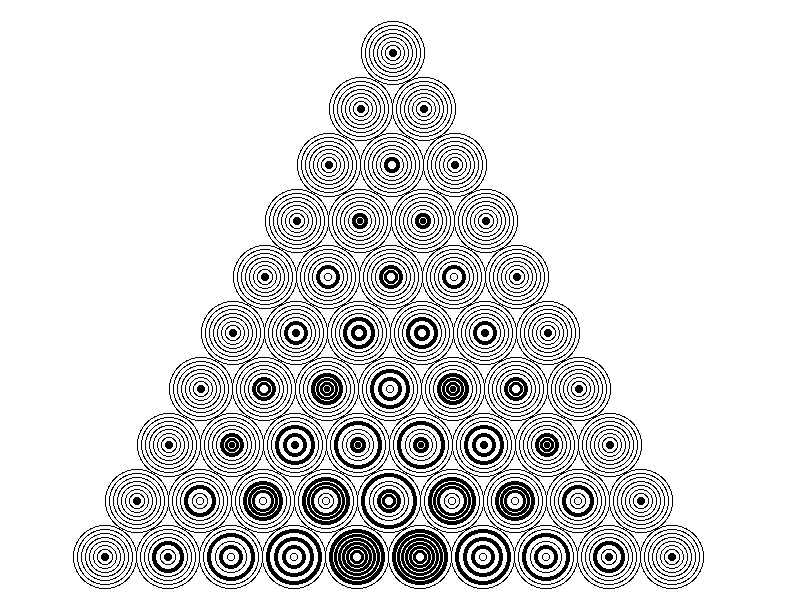# $\pi$ (...) something!

Algebra Level 5If $\pi(n)$ denotes product of all binomial coefficients in $\left(1+ x\right)^n$, then the ratio of $\pi(2002)$ to $\pi(2001)$ can be expressed as

$\dfrac{2002^{m}}{n!},$

where $m$ and $n$ are positive integers. Fin the minimum value of $m+n$.

###### Image Credit: Wikimedia Pascal Triangle by Dohduhdah
×

Problem Loading...

Note Loading...

Set Loading...